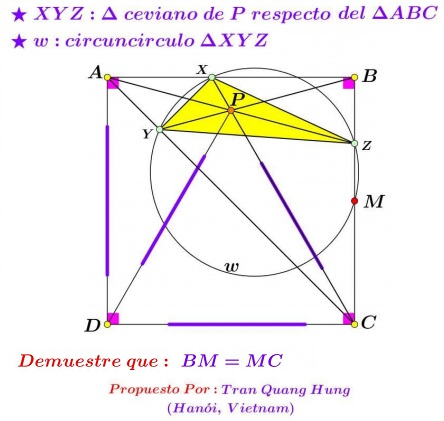# Equilateral Triangle in Square with the Cevians through Its Apex

### Source### Problem### Solution

Choose $A=-1+2i,\,$ $B=1+2i,\,$ $C=1,\,$ $D=-1.\,$ Then $P=i\sqrt{3}\,$ and we expect $M=1+i.\,$ Use the barycentric coordinates. From $P=\alpha A+\beta B+\gamma C=1\,$ and $\alpha+\beta+\gamma=1,\,$ we find $\displaystyle \alpha=\frac{1}{2},\,$ $\displaystyle \beta=\frac{\sqrt{3}-1}{2},\,$ $\displaystyle \gamma=\frac{2-\sqrt{3}}{2}.$

We have $\displaystyle \frac{B-Z}{C-Z}=-\frac{\gamma}{\beta}\,$ and, since $Z\,$ is on the line $x=1,\,$ $Z=1+2(\sqrt{3}-1)i.$

Similarly, $\displaystyle X=-\frac{2-\sqrt{3}}{\sqrt{3}}+2i\,$ and $\displaystyle Y=-\frac{1}{\sqrt{3}}+\left(\frac{\sqrt{3}+1}{\sqrt{3}}\right)i.\,$ Suffice it to show that $\displaystyle \frac{Z-M}{Z-X}\cdot\frac{Y-X}{Y-M}\,$ is real. Which is equivalent to having the following expressions real:

\displaystyle \begin{align} &\frac\sqrt{3}(2-\sqrt{3})i}2\left(\frac{1}{\sqrt{3}}-(2-\sqrt{3})i\right)}\cdot\frac-\left(\frac{\sqrt{3}-1}{\sqrt{3}}\right)(1+i)}\frac{1}{\sqrt{3}}(-(\sqrt{3}+1)+i)}\\ &\qquad\qquad\qquad=\fraci}\frac{1}{\sqrt{3}}-(2-\sqrt{3})i}\cdot\frac{1+i}{-(\sqrt{3}+1)+i}\\ &\qquad\qquad\qquad=\frac-1+i}\left(\frac{4-\sqrt{3}}{\sqrt{3}}\right)(-1+i)}\\ &\qquad\qquad\qquad=\frac1}\frac{4-\sqrt{3}}{\sqrt{3}}} \end{align

which is clearly real.

### Acknowledgment

I am grateful to Leo Giugiuc who supplied the solution and a link to the original post at the Peru Geometrico facebook group of the problem by Tran Quang Hung.